﻿ Lattice Boltzmann Simulation of Mixed Convection Flow in a Lid-Driven Triangular Cavity Subjected to Sinusoidal Thermal Forcing Utilizing NanofluidAmerican Journal of Fluid Dynamics

p-ISSN: 2168-4707    e-ISSN: 2168-4715

2018;  8(3): 84-95

doi:10.5923/j.ajfd.20180803.02### Lattice Boltzmann Simulation of Mixed Convection Flow in a Lid-Driven Triangular Cavity Subjected to Sinusoidal Thermal Forcing Utilizing Nanofluid

Atta Sojoudi

Department of Mechanical Engineering, College of Engineering, University of Tehran, Tehran, Iran

Correspondence to: Atta Sojoudi, Department of Mechanical Engineering, College of Engineering, University of Tehran, Tehran, Iran.
 Email: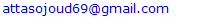Abstract

Mathematical modeling was performed to simulate mixed convection of Al2O3-Water nanofluid in a lid-driven triangular cavity employing the Lattice Boltzmann Method (LBM). Two inclined cold walls are kept at constant temperatures where the bottom lid-driven wall is subjected to sinusoidal thermal boundary condition. Different thermal conductivity and viscosity of working nanofluid were considered. The study was carried out for different Richardson numbers, 0.01≤Ri≤100, Aspect Ratios, 0.2≤AR≤1, nanoparticle solid volume fractions, 1%≤φ≤5% and different frequencies and amplitudes of sinusoidal thermal forcing of lid-driven wall. Results are presented in terms of stream function and temperature contours and mean Nusselt number of all heated walls at steady state condition. To have a better insight into the fluid flow, horizontal component of fluid velocity was plotted at mid-section of the cavity for all studied cases. The grid sensitivity test was carried out and results were validated against the experimental study.

Keywords: Lattice Boltzmann Method, Mixed convection, Al2O3-Water nanofluid, Lid-driven triangular cavity

Cite this paper: Atta Sojoudi, Lattice Boltzmann Simulation of Mixed Convection Flow in a Lid-Driven Triangular Cavity Subjected to Sinusoidal Thermal Forcing Utilizing Nanofluid, American Journal of Fluid Dynamics, Vol. 8 No. 3, 2018, pp. 84-95. doi: 10.5923/j.ajfd.20180803.02.

### 1. Introduction

Although many numerical and experimental researches have been conducted to study different aspects of engineering problems [1-7] and specifically heat transfer in fluid flow [8, 9], an analytical approach was found suitable for the current study. Fluid flow and thermal performance of lid-driven cavities have received great attention from researchers for many years due to their prime importance in lubrication technologies, electronic cooling, solar collectors, food processing and nuclear reactors . Most of the working fluids employed in lid-driven cavities with horizontal , vertical  and oscillating moving walls have low thermal performance. Due to great importance of efficient cooling systems, there is a major request for better industrial design of thermal systems to maintain the temperature of heated components below the safe limits. Choi  observed that adding nanoparticles with higher thermal conductivity to the base fluid, results in nanofluids with better thermal performance and heat transfer characteristics. Hafezisefat et al.  numerically and experimentally studied the effects of nanofluid on improvement of heat transfer inside a jacket and found good agreement between both approaches. Heat transfer enhancement by adding nanoparticles into pure water for lid-driven cavities has been confirmed through recent studies . Moreover, aspect ratios of cavity, solid volume fraction and direction of moving wall have considerable effects on fluid flow and thermal performance of the enclosure. However, it should be noted that the issue of adding nanoparticles to the base fluid still remains a controversial subject, which states that the dispersion of nanoparticles in the initial base fluid may result in a less efficient thermal performance and lower thermal conductivity of the solution than expected [16, 17]. Predication of the thermal performance of nanofluids in numerical studies depends on the employed mathematical models [18, 19]. Considering limitations in the prediction of thermal performance of nanofluids using numerical approaches, these techniques are still very helpful to study this subject [20-22] and have shown good results in academic and engineering case studies.
Plenty of studies have considered natural convection in triangular enclosures; however, few studies are related to fluid flow and heat transfer phenomenon in triangular cavity filled with nanofluid. Ghasemi and Aminossadati  numerically considered the effects of Brownian motion of nanoparticles on the natural convection of a water–CuO nanofluid in a right triangular cavity with stationary walls. They reported that it was required to consider the Brownian motion of nanoparticles in the thermal performance analysis of nanofluid-filled enclosures. They also  studied mixed convection of water-Al2O3 nanofluid in a lid-driven right triangular cavity and reported the enhancement of heat transfer rate after adding Al2O3 nanoparticles. In their other study on natural convection of Ethylene Glycol-copper nanofluid in an isosceles triangular enclosure with heat source located at its bottom wall , they found that the thermal performance of the cavity was improved with an increase in the Rayleigh number and solid volume fraction.
Commercial CFD codes are appropriate for numerical modeling of fluids; however, finite element method, using multiphysics software which offer a variety of user friendly subroutines [26, 27] can also be used to study this phenomena. Ching et al.  used finite element technique to simulate mixed convection heat and mass transfer in a right triangular enclosure. They showed that the increase of buoyancy ratio enhances the heat and mass transfer rate for all values of Richardson number and for all directions of the sliding wall motion. However, the direction of the sliding wall motion can be an appropriate control parameter for the flow and temperature fields. Rahman et al.  considered unsteady natural convection of water-Al2O3 nanofluid in a triangular enclosure where the bottom of the isosceles triangular cavity is heated non-uniformly and temperature of the inclined wall is lower than that of the bottom wall. They concluded that heat transfer increases with addition of nanoparticle and increase in Rayleigh number.
To the best of the knowledge of the authors, very limited studies are related to the natural convection of nanofluid in a triangular enclosure subjected to the sinusoidal thermal forcing. In the present study, thermal performance of a lid-driven triangular enclosure filled with water-Al2O3 nanofluid where the bottom driven wall is subjected to the sinusoidal thermal forcing is determined.

### 2. Mathematical Model and Simulation Methodology

#### 2.1. Physical Properties of Nanofluid

Density of the water-Al2O3 nanofluid is calculated through the classical formula developed for conventional solid-liquid mixtures: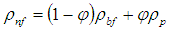(1)
where φ indicates volume fraction of nanoparticles and subscripts p, bf and nf represent the particle, base fluid and nanofluid, respectively. By considering a thermal equilibrium between the particles and the surrounding base fluid, specific heat is calculated as follow: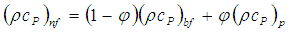(2)
The thermal expansion coefficient is evaluated by: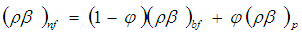(3)
And thermal diffusivity of the fluid is defined as: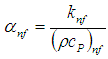(4)
where thermal conductivity of the nanofluid was calculated by the Chon et al. model  by: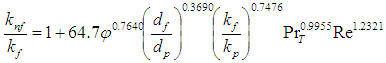(5)
PrT and Re are introduced by: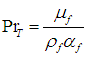(6)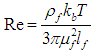(7)
The symbol kb is the Boltzmann constant =1.3807×10-23 J/K, and lf is the mean path of fluid particles given as 0.17nm . This model is based on experimental measurements of water-Al2O3 nanofluid  in accordance to Minsta et al. tests , it is suitable to predict the effective thermal conductivity up to a volume fraction of 9% and temperature range of 21°C to 70°C. For nanofluid flow, the particle Knudsen number is very small and is around 0.003.
Nguyen et al.  reported experimental results on water-Al2O3 nanofluid dynamic viscosity, but since their results didn't have any explicit correlation to define the viscosity of the nanofluid in terms of the both temperature and solid volume fraction, Abu-Nada et al.  introduced a new correlation including both parameters as below: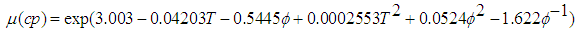(8)
where the viscosity is expressed in centi poise and the temperature in °C. Thermophysical properties of the solid particle and the base fluid are shown in Table 1.
 Table 1. Thermophysical properties of fluid and nanoparticles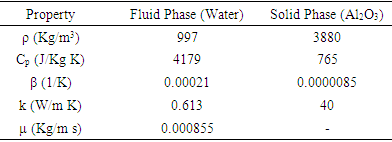#### 2.2. Governing Equations

Natural convection heat transfer of water-Al2O3 nanofluid in a two dimensional lid-driven triangular cavity is considered (Fig. 1). H and L are height and length of the base of the triangle, respectively. Inclined cold walls are kept at Tc while the bottom driven wall is sinusoidally heated. A is thermal forcing amplitude where 0.5n shows its frequency. For n=2, there is a complete cycle of thermal heating, in other words, half of the base is heated to Th and the other half is cooled to Tc. For Steady state condition, laminar, incompressible and homogeneous Newtonian fluid is assumed for the present study. Governing equations for continuity, momentum and fluid energy are defined as follow: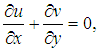(9)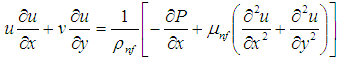(10)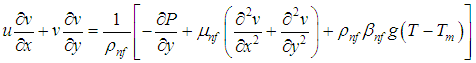(11)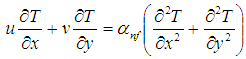(12)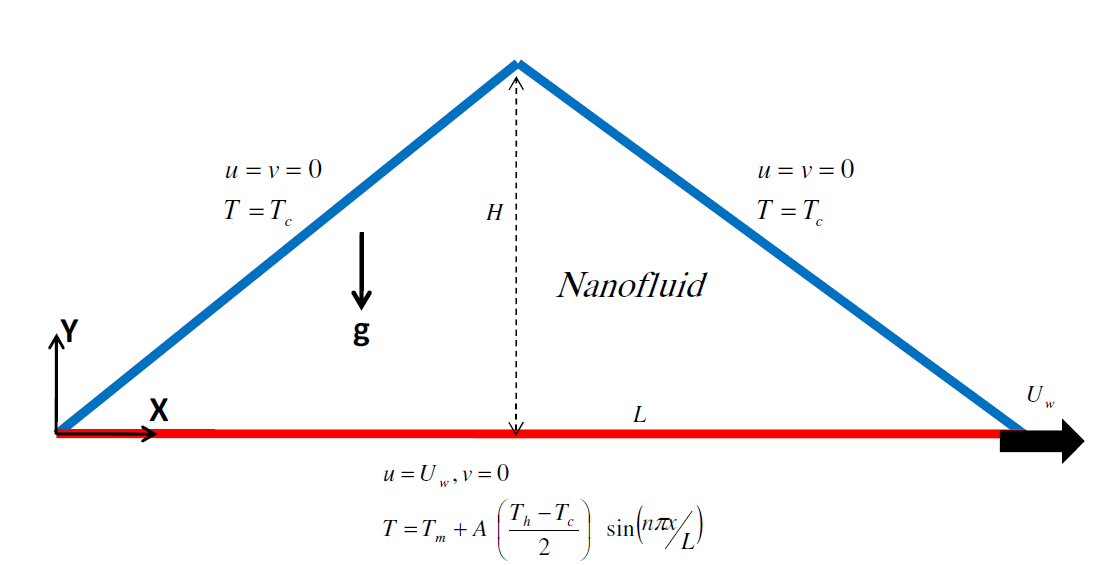Figure 1. A schematic diagram of the physical model
Boundary conditions are: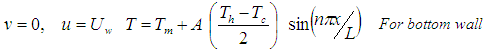(13)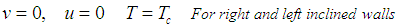(14)
Non-dimensional controlling parameters of the problem are given as below: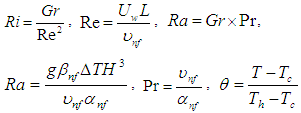Two important parameters of local Nu and mean Nu are defined below, and are widely investigated.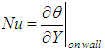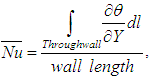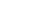l is parallel to the wall

#### 2.3. Lattice Boltzmann Method

Since the Lattice Boltzmann Method (LBM) is much more computationally efficient than classical CFD [34-38] or image processing techniques [39-41], it was used to determine the desired flow field and heat transfer phenomenon; where it adopts a uniform lattice with BGK collision model. Thermal lattice Boltzmann equations  are remarked as follow: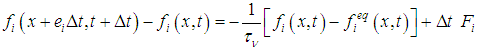(15)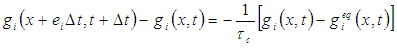(16)
where ei represents particle velocity direction and fi and gi show particle density and energy distribution functions, respectively. τv and τc express dimensionless relaxation times that controls the rate of approaching equilibrium.
D2Q9 model  is utilized for the current 2D application. In this model discrete velocities are introduced as follows: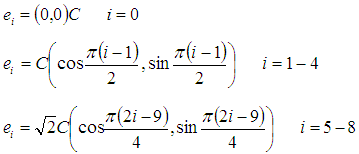(17)
The equilibrium density distribution function is: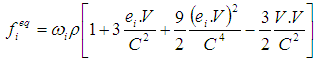(18)
where the weighting factors ωi are ω0=4/9, ωi=1-4=1/9 and ωi=5-8=1/36, and C is assigned as lattice speed and given by: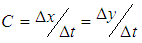(19)
where ∆x and ∆y are grid spacings in x and y direction, while ∆t is the discrete time step.
The equilibrium energy distribution functions are given as: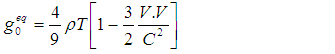(20)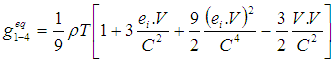(21)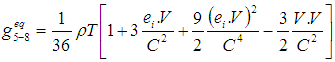(22)
Macroscopic variables are evaluated as follows: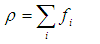(23)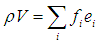(24)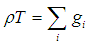(25)
It has been demonstrated that evolution of Eqs. (15-16) can retrieve the macroscopic Eqs. (9-12) . Boussinesq approximation, buoyancy term is supposed to be linearly dependent on the temperature as follow: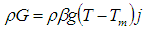(26)
where g is gravitational acceleration, Tm=(Th+Tc)/2 and j represents direction opposite to that of gravity. Fi is added for Eq. (15) to account for the buoyancy term and is given as follow: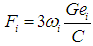(27)
Two step procedure, namely collision step followed by a streaming step is used to solve the density and energy distribution functions where this manner requires computational effort since it develops the data from neighboring lattice points, while collision step is completely localized.

#### 2.4. Convergence Criterion

A uniform grid was used for all simulations where the convergence criteria is defined as: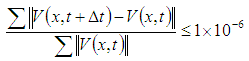(27)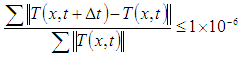(28)

### 3. Results and Discussion

In the current study, it was assumed that Ra=105. The Richardson number (Ri), and solid volume fraction (ϕ) were considered to be in the range of 0.01≤Ri≤100 and 0≤ϕ≤0.05, respectively. The analysis was performed for pure water (ϕ=0), as well as for a water-based nanofluid with 38 nm diameter Al2O3 nanoparticles.

#### 3.1. Simulation Validation

Numerical simulations were performed using different mesh sizes ranging from 10000 up to 17000. A FORTRAN code is developed to solve the present problem using a structured mesh of square type. The details of this method are mentioned in references [42, 43]. It was concluded that the optimal computational mesh that contributed grid independent solutions comprised of 15600 elements. Symmetrical lattices with respect to the midplane of the triangular enclosure were distributed.
For validation of numerical results, static and adiabatic thermal boundary conditions for bottom wall, and differential heating for the right and left walls were assumed. Considering a triangular enclosure of AR=1 with Gr=2.1577×107 and Pr = 0.71, numerical results of local Nu for cold inclined wall were compared with Flack et al. . Fig. 2 shows a satisfactory agreement between present results and the previous results from the experimental study of Flack et al .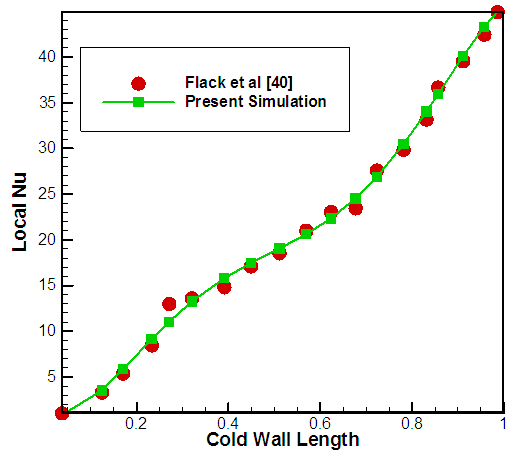Figure 2. Comparison of local Nu for cold inclined wall with Flack et al 
Next section of this paper deals with transient flow development, steady state results and heat transfer phenomena of the natural convection within triangular enclosure with deferentially heated inclined walls and bottom heat source with highly conductive partition installed at the middle of the region.

#### 3.2. Effect of Ri

In this section, effect of Ri on flow field and heat transfer phenomenon is studied. Fig. 3 illustrates contours of temperature and stream function for water-Al2O3 nanofluid with 2% solid particle volume fraction, AR=0.5, A=1 and n=2. A large cell is formed near the right corner, due to the movement of bottom wall for Ri= 0.01. With increase in Ri to 0.1, single cell is shifted toward the center of the cavity, later divided into two cells near the bottom wall due to the strengthening buoyant flow. It is evident that maximum stream function value is reduced with the increase of Ri. For higher value of Ri, Ri=10 and 100, one large cell is formed at the core of the enclosure approximately occupying the entire domain and gets lager with increase in Ri, while a little cell that is created at the right corner of the cavity gets smaller due to the development of large central cell. From the temperature contours, it is evident that for lower Ri, due to dominant forced convection heat transfer, core and upper part of cavity are influenced by high temperature of half bottom wall. With increase in Ri, natural convection heat transfer is dominant and nearly left half of the enclosure is effected by left bottom hot part, and right half of the cavity is influenced by right cold bottom part.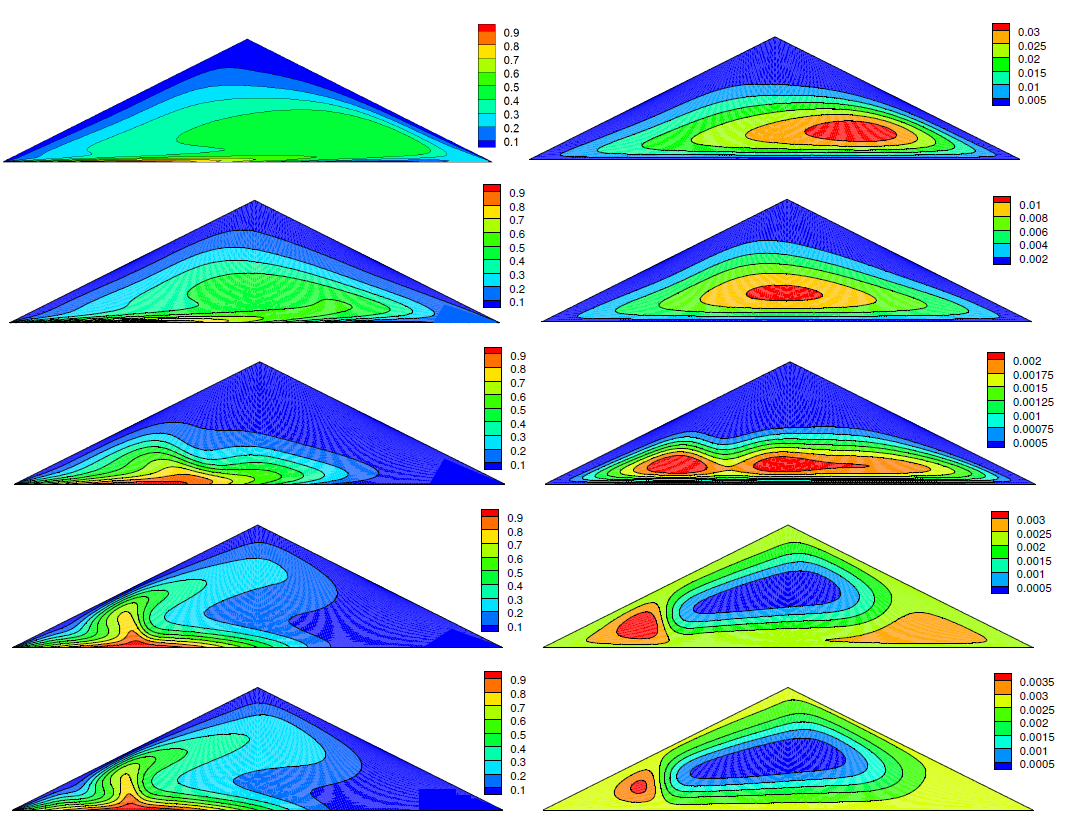Figure 3. Dimensionless temperature (Left) and stream function (Right, Kg/s) contours of water-Al2O3 nanofluid with φ=2%, AR=0.5, A=1 and n=2
To have a better insight into the flow field of nanofluid filled enclosure, profiles of x-velocity (U) at the midsection of the cavity are plotted in Fig. 4 for φ=2%, AR=0.5, A=1 and n=2. Results generally demonstrate that the absolute magnitude of U increases as Ri is increased. This is due to the strengthening of the buoyant flow field at higher Ri values.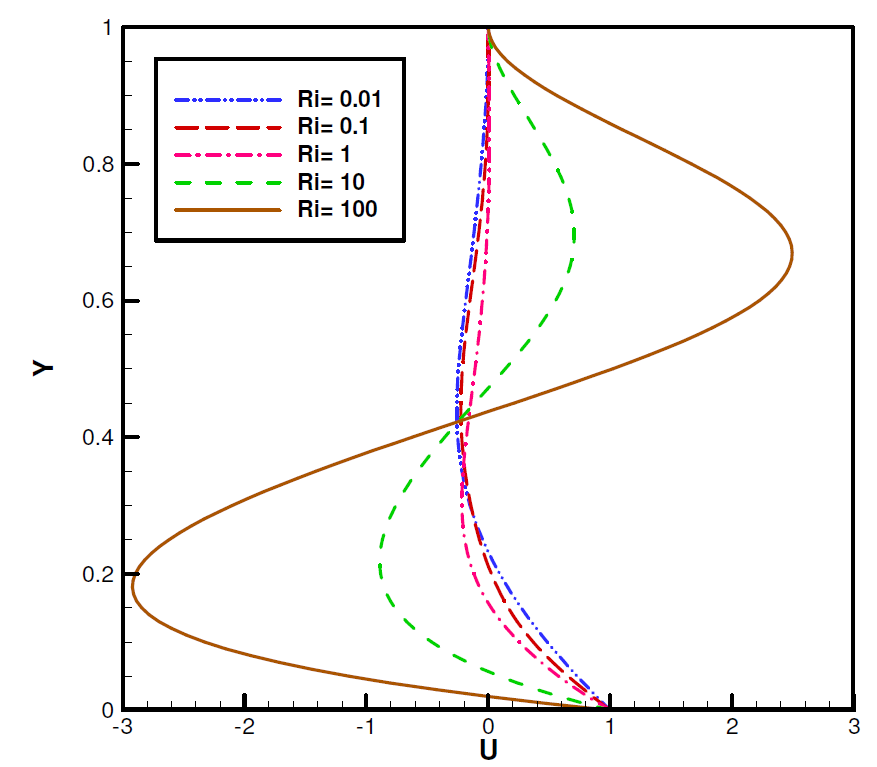Figure 4. Profiles of x-velocity (U) at the midsection of the cavity for =2%, AR=0.5, A=1 and n=2
Fig. 5 presents mean Nu variation of three walls in terms of Ri for φ=2%, AR=0.5, A=1 and n=2. It is shown that for moving wall, mean Nu is decreases with increase in Ri up to Ri=1, due to the descending magnitude of force convection heat transfer, and later it rises up on behalf of reinforced natural convection effect. Much of the heat is regressed from the left cold wall by increase in Ri, and this is attributed to the fact that, higher Ri results in dominant natural convection heat transfer for the left portion of the bottom wall and left cold inclined wall?. So we should expect that less heat will leave the thermal system through right cold wall, because there is lower temperature difference between the right portion of the bottom wall and the right cold inclined wall.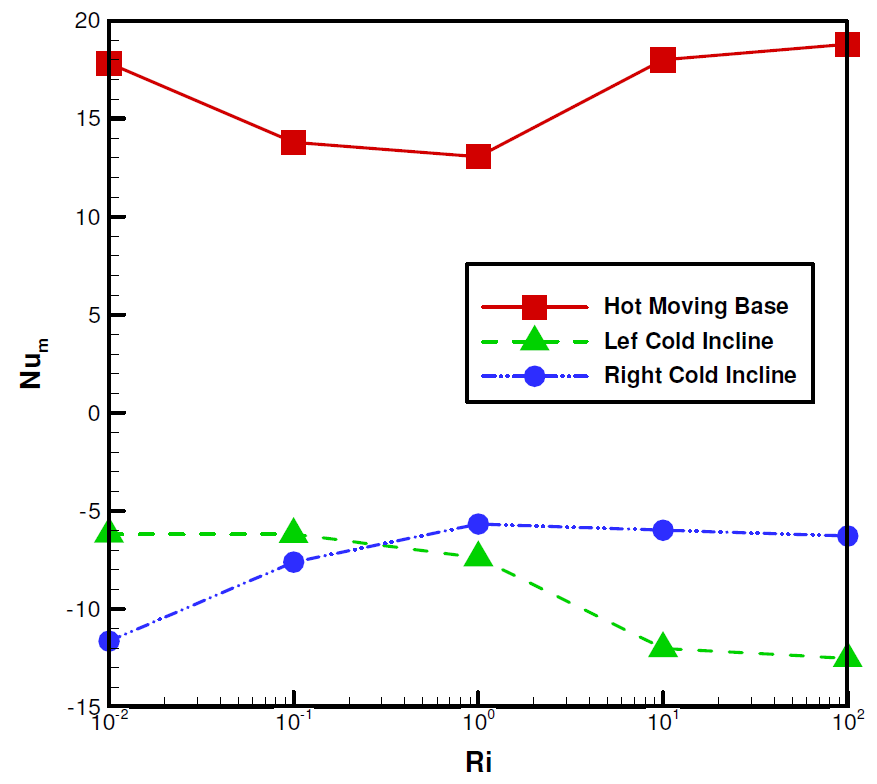Figure 5. Mean Nu variation of three walls in terms of Ri for =2%, AR=0.5, A=1 and n=2

#### 3.3. Effect of AR

Fig. 6 shows the effect of different AR on distribution of temperature and stream function for φ=2%, Ri=1, A=1 and n=2. For AR=0.2, due to the lower distance of the left cold inclined wall and the left hot portion of the bottom wall, conduction heat transfer is dominant for the left corner, while the natural convection heat transfer is dominant for middle part of the cavity. Three cells are formed within the cavity. With increase in AR, number of cells are reduced and forced convection heat transfer is reinforced, so more than half of the enclosure is affected by the bottom high temperature. There is only one cell at the core of enclosure for AR=1. U velocity profiles at the midsection for three different ARs are plotted in Fig. 7 for Ri=1. It is seen that absolute magnitude of horizontal velocity is higher for lower AR due to the dominant natural convection heat transfer for the middle part of the cavity. Fig. 8 represents mean Nu of three walls of the cavity for various AR, φ=2%, Ri=0.01 and 100, A=1 and n=2. It is seen that with increase in AR, mean Nu of the bottom wall is reduced, which is related to reduction of shear stress according to Fig. 7. Absolute value of mean Nu for inclined walls are also reduced with increase of AR. It is due to the reduction of heat flux into the system from the bottom wall.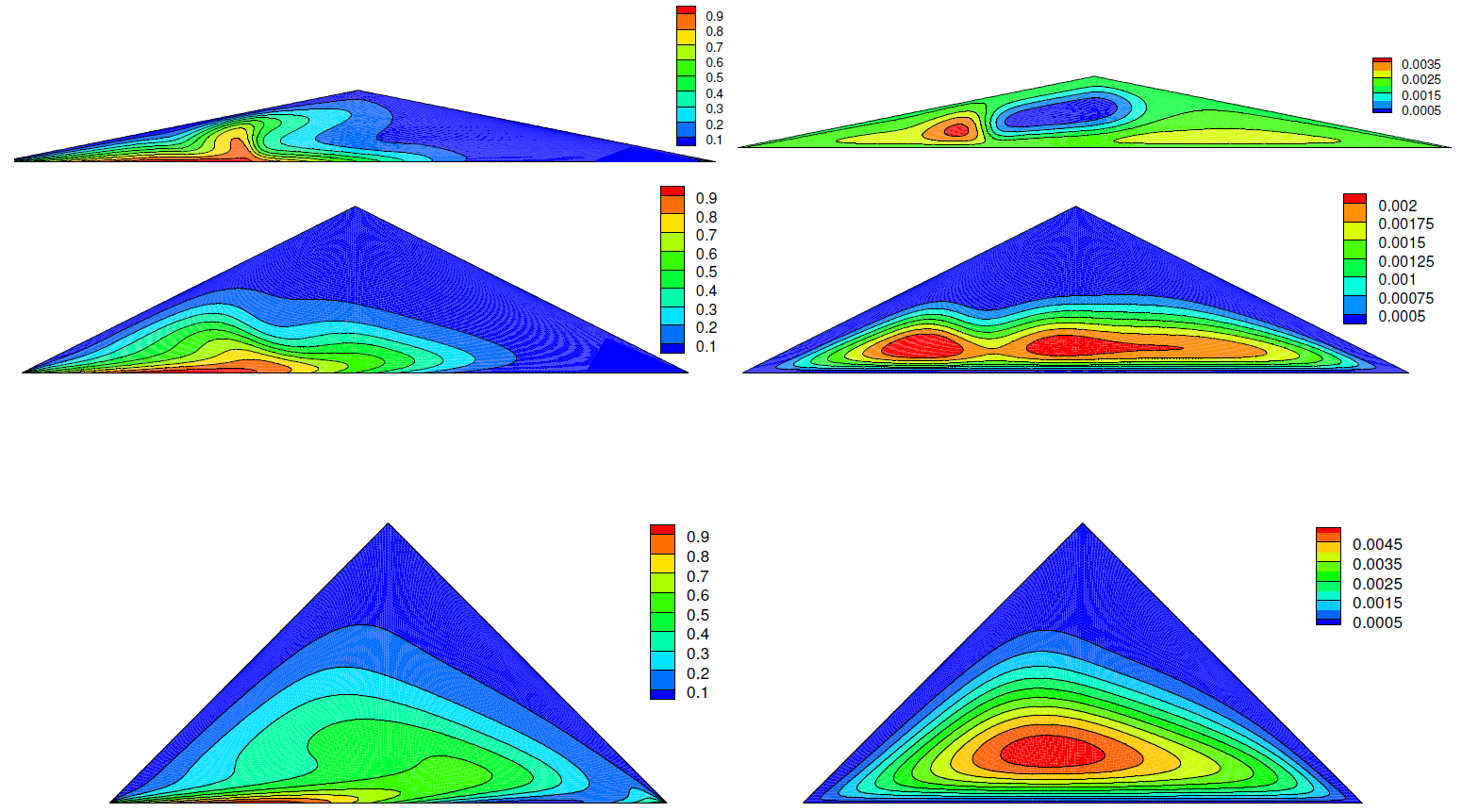Figure 6. Dimensionless temperature (Left) and stream function (Right, Kg/s) contours for various AR when φ =2%, Ri=1, A=1 and n=2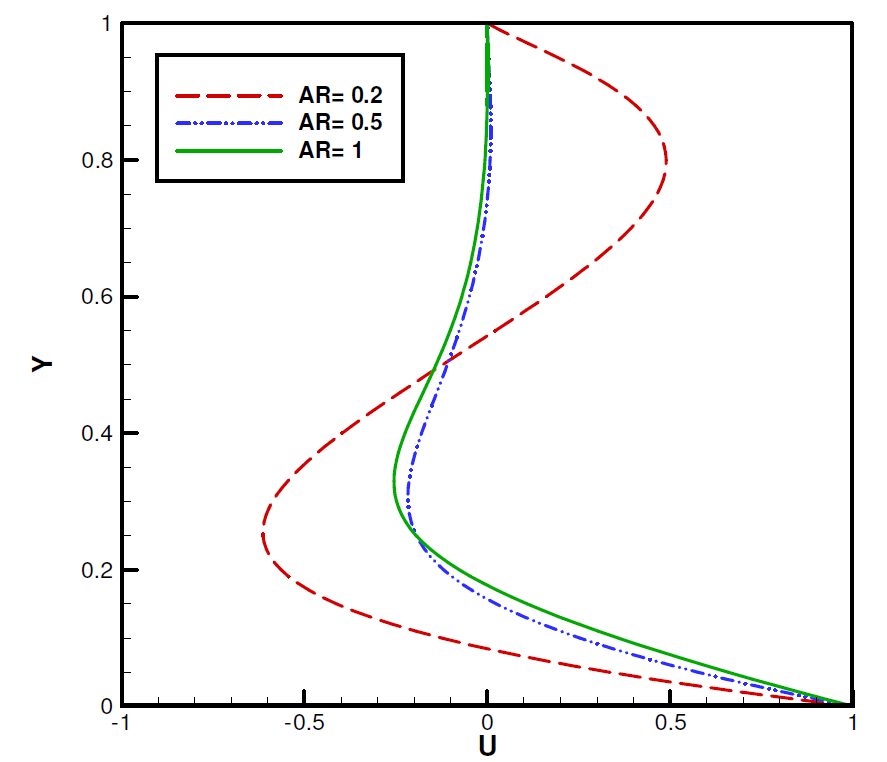Figure 7. Profiles of x-velocity (U) at the midsection of the cavity for different AR and φ =2%, Ri=1, A=1 and n=2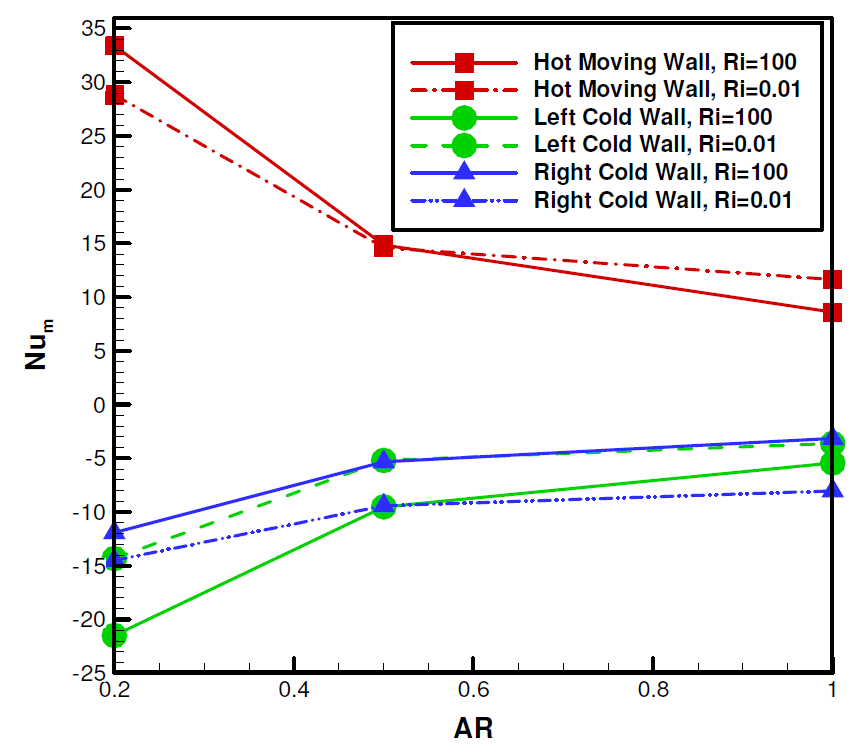Figure 8. Mean Nu variation of three walls in terms of AR for φ =2%, Ri=0.01 and 100, A=1 and n=2

#### 3.4. Effect of Nanofluid Volume Fraction

In Fig. 9, contours of temperature and stream function for various solid volume fractions of water-Al2O3 nanofluid are plotted while AR=0.5, Ri=1, A=1 and n=2. It is evident that for φ=1%, two rotating cells are formed near the bottom wall. With increase in solid volume fraction, natural convection heat transfer becomes dominant where 3 rotating cells are formed for φ=5%, and maximum stream function is enhanced up to higher solid volume fraction. U velocity profiles of different solid volume fractions are plotted in Fig. 10. There is a similar flow pattern and approximately equal U magnitude for all solid volume fractions at the same Ri, and the existing difference of the magnitude of U when φ=3% at Ri=100 may be related to the displacement of the cells that are formed near the bottom wall. Fig. 11 displays mean Nu of walls in terms of solid volume fractions. The absolute heat transfer rate increases as the solid volume fraction increases. This is due to the increase in the volume of solid nanoparticles with relatively higher thermal conductivity. It is also noteworthy that the right cold inclined wall is associated with higher absolute mean Nu for lower Ri, while the left cold wall has higher absolute mean Nu for higher values of Ri (natural convection is dominant).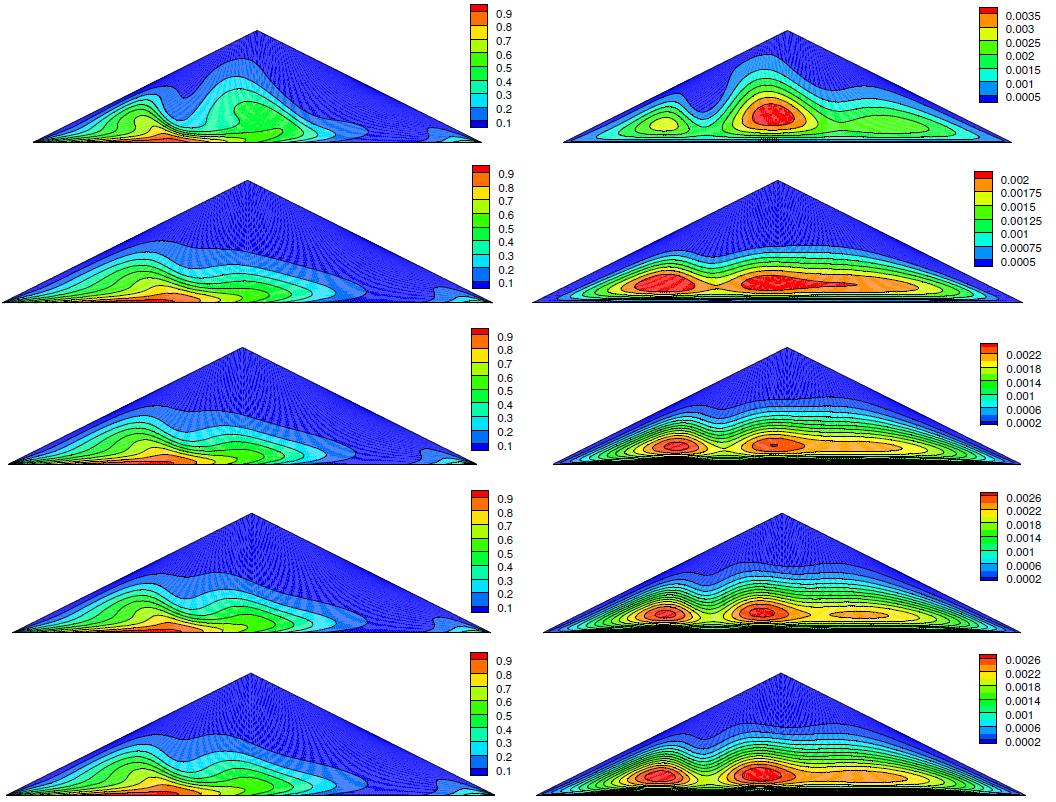Figure 9. Dimensionless temperature (Left) and stream function (Right, Kg/s) contours for various solid volume fractions of water-Al2O3 when AR=0.5, Ri=1, A=1 and n=2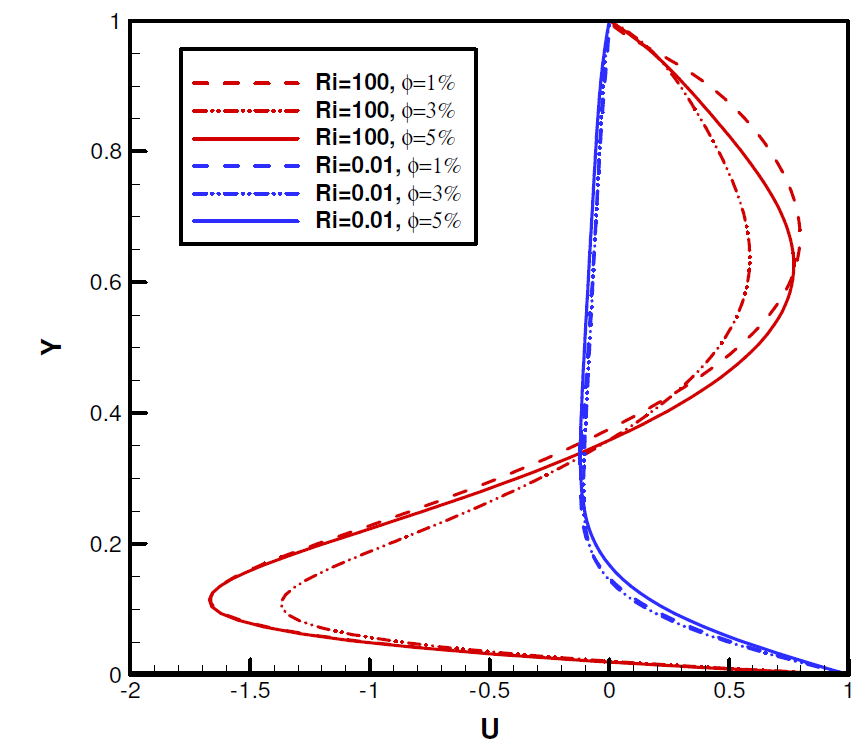Figure 10. Profiles of x-velocity (U) at the midsection of the cavity for different solid volume fractions and AR=0.5, Ri=0.01 and 100, A=1 and n=2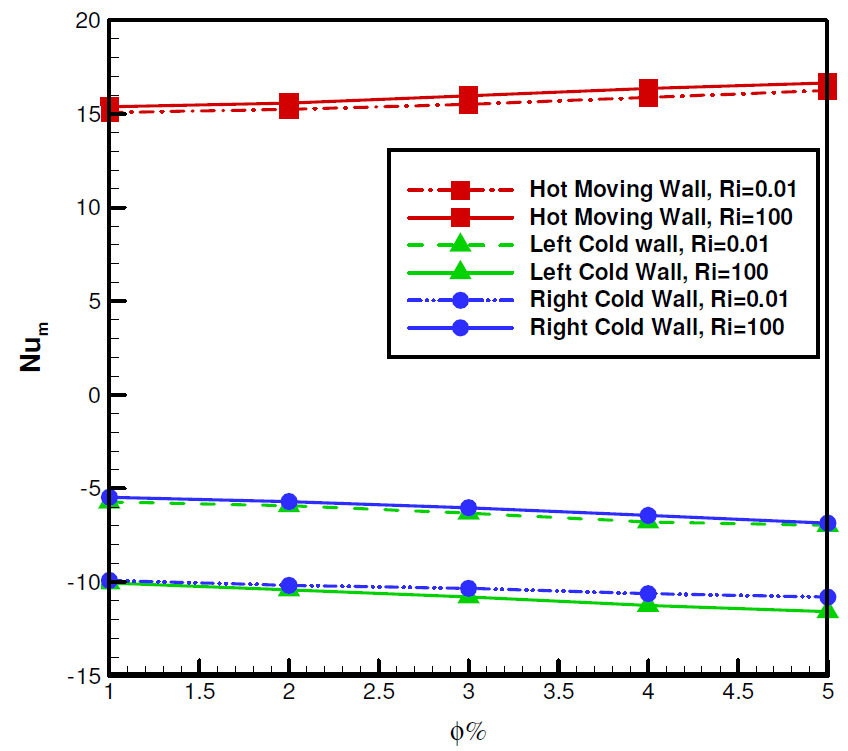Figure 11. Mean Nu variation of three walls in terms of solid volume fractions for AR=0.5, Ri=0.01 and 100, A=1 and n=2

#### 3.5. Effect of Frequency and Amplitude of Thermal Forcing

In this section, effects of frequency and amplitude of sinusoidal thermal forcing on flow field and heat transfer is discussed. Fig. 12-a displays contours of temperature and stream function for various thermal forcing frequency when AR=0.5, φ =2%, Ri=1, A=1. Maximum value of stream function is increased with the enhancement of the frequency. Moreover, cellular flow pattern is seen where 2 rotating cells are formed near the bottom wall and a single cell is created near the top. Much of the area of the enclosure is affected by the hot temperature of the bottom wall for higher frequencies. In Fig. 12-b, it is illustrated that for A=0.5, stratified temperature contour occupies the locations adjacent to the bottom wall and with increase in amplitude more cells are formed within the cavity. Parallel stream lines form adjacent to the inclined walls for A=1.5, which are associated with strong conduction heat transfer mechanism.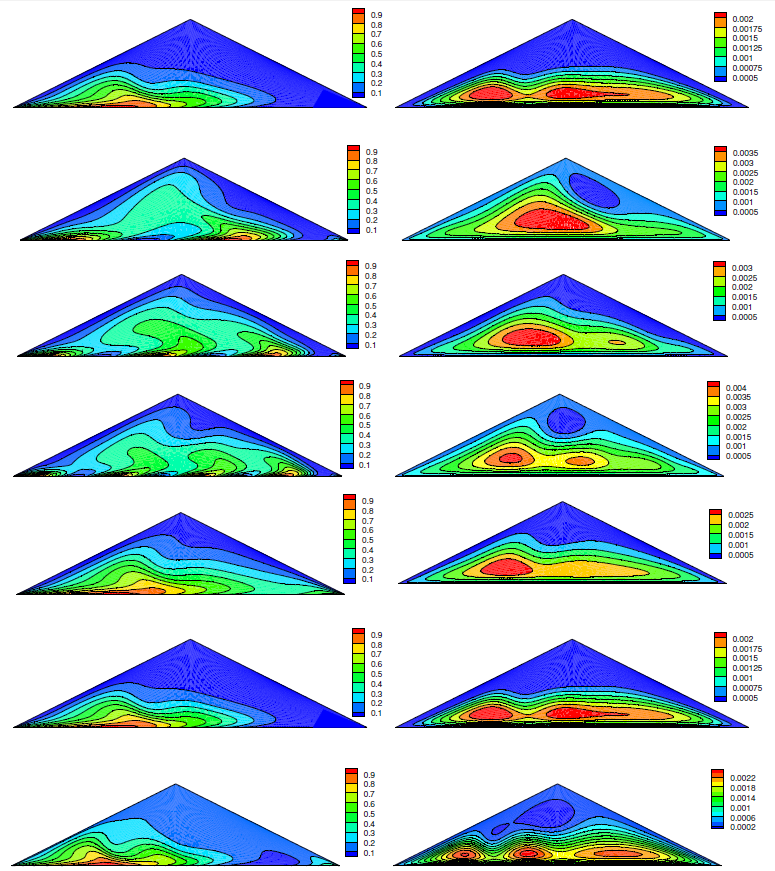Figure 12. Dimensionless temperature (Left) and stream function (Right, Kg/s) contours for a) Frequency (1 to 4) b) Amplitude (0.5 to 1.5) of water-Al2O3 when AR=0.5, φ=2% and Ri=1
Fig. 13 displays U velocity profiles for different frequencies and amplitudes. It is seen that absolute magnitude of x velocity is higher for higher frequencies and its magnitude is equal for all amplitudes, while the maximum U velocity is displaced. Fig. 14 displays mean Nu of walls for different frequencies and amplitudes when AR=0.5, φ=2%, Ri=1. As seen in Fig. 14-a, absolute mean Nu is increased for higher frequencies. For the moving wall, a slight decrease and then an increase of mean Nu is seen with the increase in frequency and for higher Ri that are associated with higher mean Nu of the bottom wall. Fig. 14-b shows mean Nu variation for different amplitudes. In this figure, mean Nu differs for various amplitudes. It can be concluded that these variables (frequency and amplitude) can be considered as suitable controlling parameters for heat transfer phenomenon within the cavity.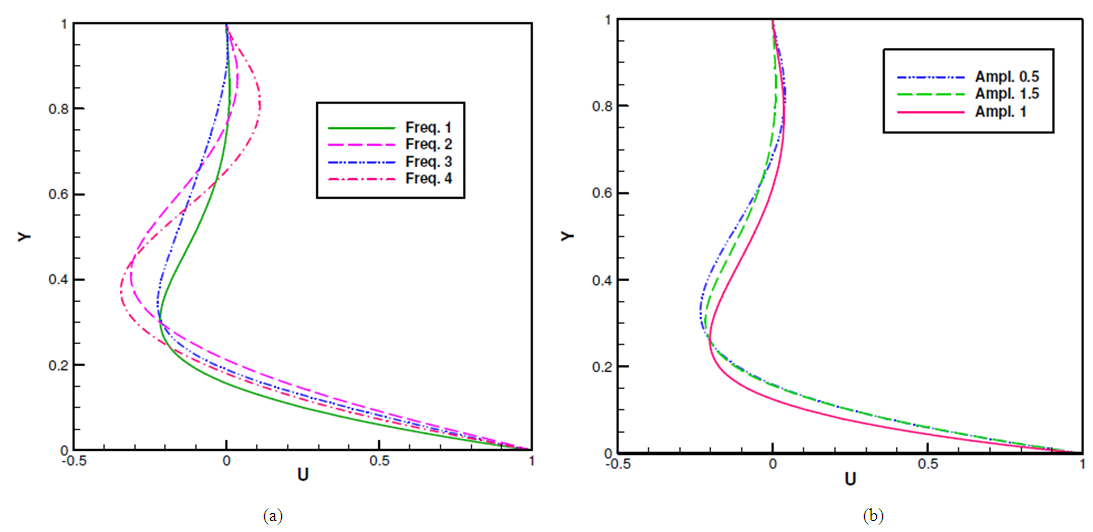Figure 13. Profiles of x-velocity (U) at the midsection of the cavity for a) Frequencies b) Amplitudes and φ =2%, AR=0.5, Ri=0.01 and 100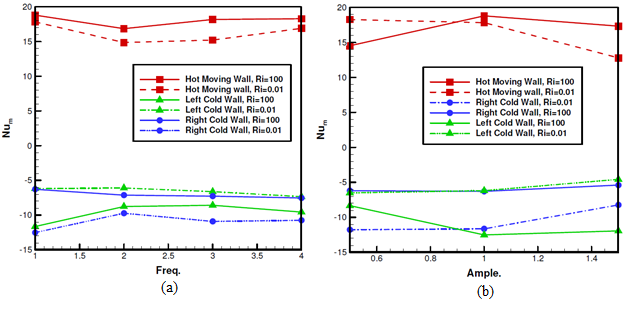Figure 14. Mean Nu variation of three walls in terms of a) Frequency b) Amplitude for AR=0.5, Ri=0.01 and 100

### 4. Conclusions

The natural convective flow and heat transfer features of a lid-driven triangular enclosure with 38nm Al2O3/water nanofluids are numerically tested using the lattice Boltzmann method (LBM). Incline boundaries of the cavity are cooled to a constant temperature while the bottom driven wall is subjected to a sinusoidal thermal forcing. Using variable viscosity and thermal conductivity models, the analysis was performed for different Richardson numbers, 0.01≤Ri≤100, Aspect Ratios, 0.2≤AR≤1, nanoparticle solid volume fractions, 1%≤φ≤5% and different frequencies and amplitudes of sinusoidal thermal forcing of lid-driven wall. Some important outcomes are as follow:
• The absolute magnitude of U increases as Ri increases.
• For the moving wall, mean Nu decreases with increase in Ri up to Ri=1, due to the descending magnitude of force convection heat transfer, then it rises up on behalf of reinforced natural convection effect.
• With increase in AR, number of cells are reduced and forced convection heat transfer is reinforced.
• With increase in AR, mean Nu of the bottom wall is reduced; this is related to the reduction of shear stress near the moving wall.
• Addition of nanoparticles showed to improve the heat transfer rate in the cavity.
• Variation of frequencies and amplitudes of sinusoidal thermal forcing affects the flow and temperature distribution within the enclosure.